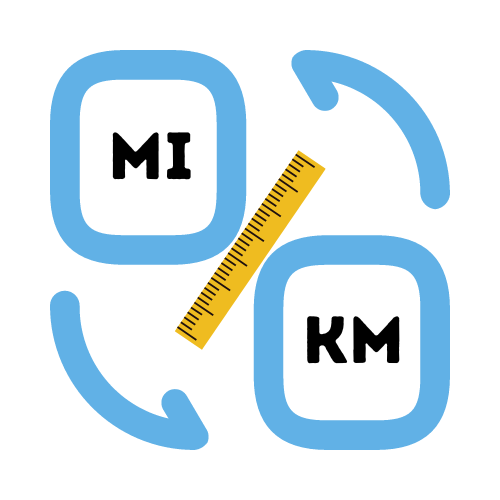# Convert Miles to Kilometers

## Simple conversion of miles to km.

Enter distance in miles

Enter a number.

Total distance:

Distance is one of the most important measurements in the world. How you measure distance often depends on where you are in the world. Some countries use kilometers, while other countries measure distance in miles.

There will be times when you need to know how to convert between kilometers and miles. How many kilometers are in a mile? How far is that in kilometers? How do you convert between the two?

## How do you convert miles to kilometers?

One of the most common units of length is the mile. It is used in most countries including the United States, Canada and the United Kingdom. It is a standard US Customary Measure unit and is equivalent to 5,280 feet, or 1,609.344 meters.

If you've spent any time overseas then you will likely have asked yourself the question at some point. How do you change miles to kilometers? Most people struggle to interchange values between miles and kilometers, and because of that they don't know how many miles to the kilometer.

Each mile is equal to 1.60934 kilometers, and each kilometer is equal to 0.6214 miles. Knowing the equivalent distance for each measurement makes the calculation very simple. For example: 10 miles to kilometers can be calculated as follows:
`10 * 1.60934 = 16.09 km`.

## How long does it take to walk 5 miles?

Most of us can walk 3.5 miles per hour and typically cover about 17 minutes for each one mile walked or about 85 minutes over 5 miles. If you walk faster, then obviously your walking time will decrease dramatically. When walking at a pace of 4 miles per hour, one can cover each mile in 15 minutes instead while taking 1 hour and 15 minutes to complete the five mile distance in total.

## What is the formula for miles to kilometers?

Also known as "statute miles," "land miles" and "nautical miles," the mile is a unit of measurement in many different countries. The mile is used to measure how far something is from an observer. It is equal to 5,280 feet or 1,609 meters.

The mile is used most often in the United States, but is also used in Canada, the United Kingdom and a few other places where the metric system is not as widely used. One mile is equal to 1.61 kilometers. You can convert miles to km by multiplying the number of miles by 1.60934.

For example:
`100 miles * 1.60934 = 160.93km`

## How the miles to km calculator can help

Simply enter your distance in miles and the calculator will instantly convert the miles to km. We also have a separate distance calculator to convert km to miles which works in the same way.

A distance calculator is a tool that makes it easy for people to convert from miles to kilometers. These calculators are great for people who are used to thinking and working in miles, but need to convert their work for a presentation or a different purpose. Calculations are an important part of every industry and all people who are under pressure to have accurate information should be able to calculate distance and convert miles to km easily using a distance converter.